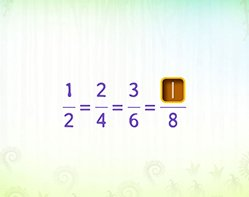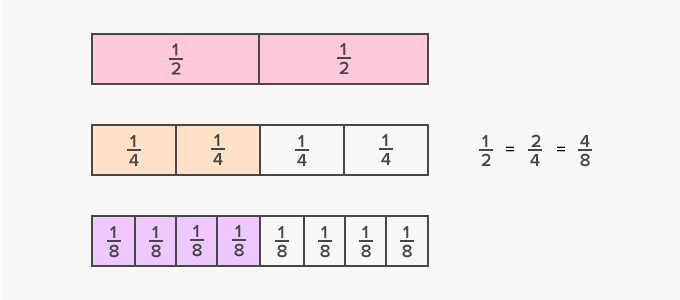# Equivalent Fractions - Definition with Examples

The Complete K-5 Math Learning Program Built for Your Child

• 30 Million Kids

Loved by kids and parent worldwide

• 50,000 Schools

Trusted by teachers across schools

• Comprehensive Curriculum

Aligned to Common Core

## Equivalent Fractions GamesEquivalent Fractions

Get comfortable with equivalent fractions. Mastering this concept will go a long way in helping you master fractions in this grade.

Covers Common Core Curriculum 4.NF.1

## What are Equivalent Fractions?

In math, equivalent fractions can be defined as fractions with different numerators and denominators that represent the same value or proportion of the whole.

Here’s an example of equivalent fractions.Fun Facts Equivalent fractions represent the same amount of distance or points on a number line.  All equivalent fractions reduce to the same fraction in their simplest form.

##Let's sing!

When carrying out operations on equivalent fractions
Change the denominator using multiply or divide,
And the same to the numerator must be applied.

##Let's do it!

Instead of handing out regular fraction worksheets to your children, ask your child to divide the pizza or pancakes such that he can show three equivalent fractions.

##Related math vocabulary

Won Numerous Awards & Honors